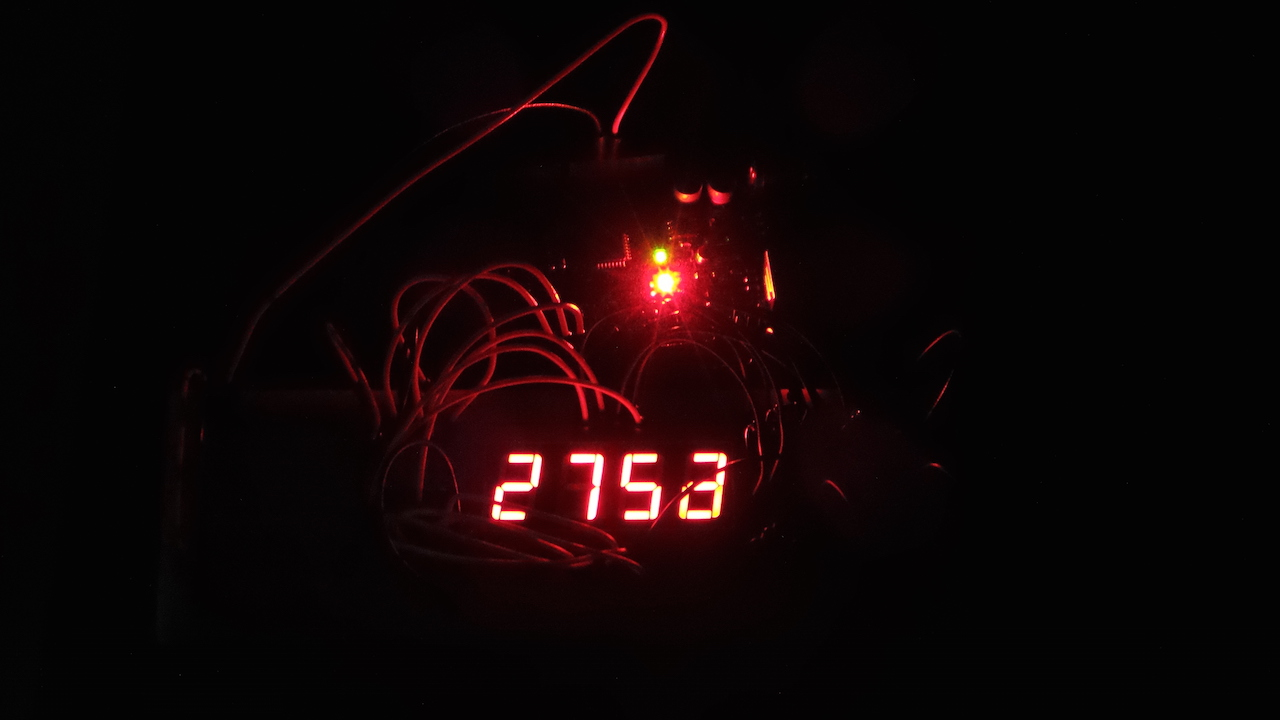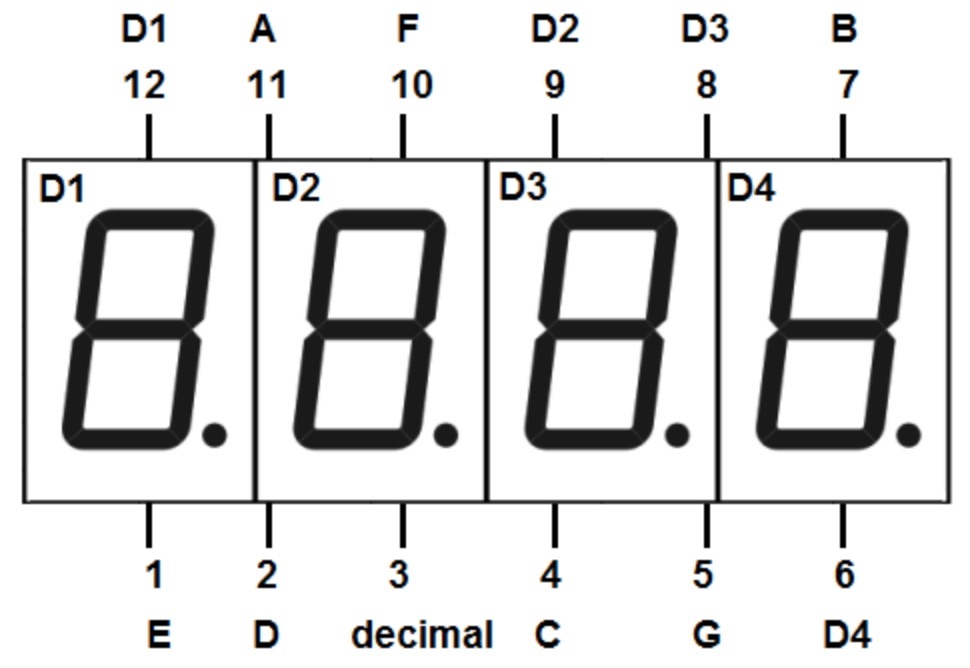# #058 Led4Digit7Segment

Driving a 4-digit 7-segment display, various ways…There are a number of similar 4-digit 7-segment LED displays in the market. Although basically the same, they vary in critical ways:

• common anode or common cathode configuration
• number and assignment of pins

The basic unit will have 12 pins, and each “digit” will comprise 7 LED segments plus a decimal point. Units are also available with 14 or 16 pins. The additional pins are used to control punctuation and other effects such as the colon typically used in 12/24 hour clock displays.

Although pin assignments vary, they all operate in a similar manner:

• a pin is common to a specific LED segment that is repeated across all digits e.g. pin 8 = “top bar of the digit”
• another pin is common to all elements in a given digit e.g. pin 6 = “all elements in the first digit”
• creating (powering) a circuit across the two pins will light the selected segment in the selected digit
• the “common anode”/”common cathode” terminology basically indicates the polarity of the circuit. Common cathode means all the LED segments share a common cathode (negative pole) and are switched on by pulling segment pins high.

### SMA420564

So much for theory; the hardest thing tends to be finding the right datasheet! I was unable to find a datasheet matching the part I have - stamped as an “SMA420564” - but figuring it out just takes a little poking around with a 5V + resistor probe. Here’s my ‘reverse-engineered’ datasheet:

It’s a 4-digit common cathode unit, with the common cathode for each digit on pins 6, 8, 9, 12.

The anode connections for each segment are mapped in the folliwng table. The LED segments are labelled clockwise from A (top bar) to F (top left bar); G is the centre bar; and ‘dp’ is the decimal point segment after the digit:

pin 12 (D1) 9 (D2) 8 (D3) 6 (D4)
1 E E E E
2 D D D D
3 dp dp dp dp
4 C C C C
5 G G G G
11 A A A A
10 F F F F
7 B B B B### Test Circuits

While you can wire up each segment pin to a digital out on the Arduino, it soon becomes pretty wasteful of pins. And how we program the unit depends in turn on how we drive it. See the following projects for various approaches:

• SingleShift - uses an 8-bit shift register and some BJTs, total of 7 Arduino GPIO pins required
• DoubleShift - uses two 8-bit shift registers and some BJTs, total of 3 Arduino GPIO pins required
• DoubleShiftWithFETs - uses two 8-bit shift registers and some n-channel FETs, total of 3 Arduino GPIO pins required